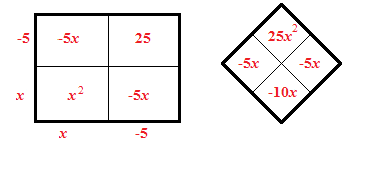### Home > INT2 > Chapter 7 > Lesson 7.1.2 > Problem7-27

7-27.

Use the Zero Product Property or complete the square to solve the following quadratic equations. Then check your solutions.

1. $x^2-10x+25=0$

Use an area model and a diamond problem to help you factor the equation.$x = 5$

1. $0=3x^2+17x-6$

See part (a).

1. $3x^2-2x=5$

Rewrite the equation so that it is equal to $0$.

$x=\frac{5}{3}$$x=-1$

1. $16x^2-9=0$

You should notice that this is a difference of squares.